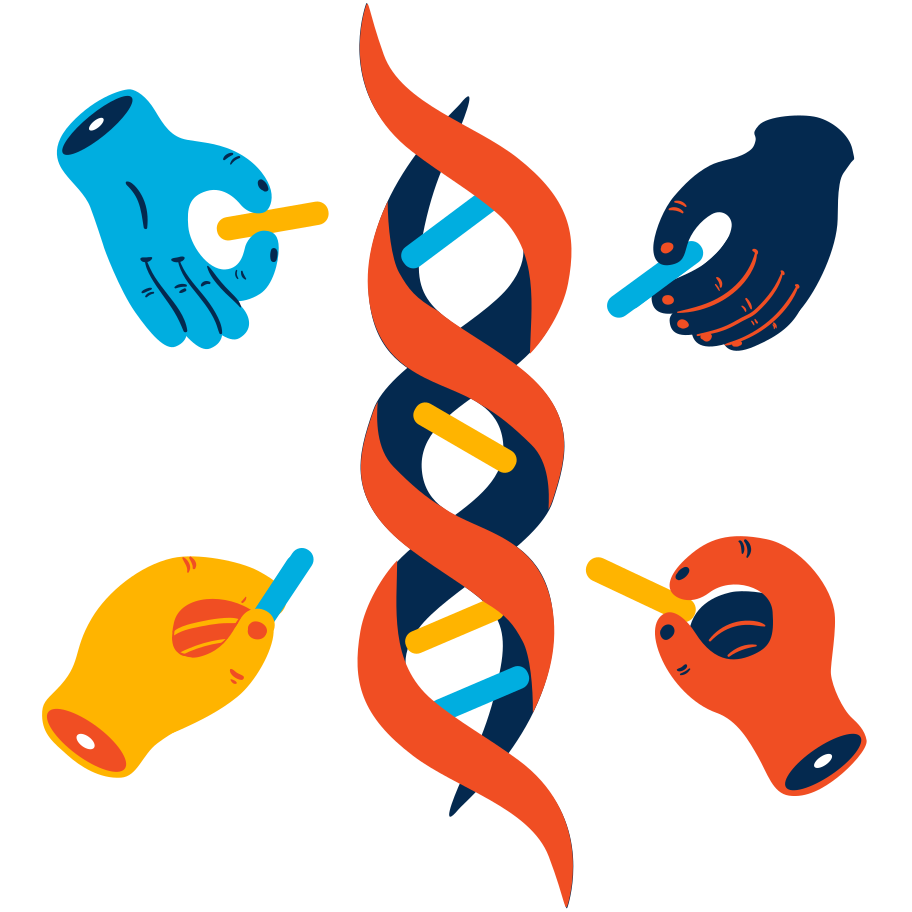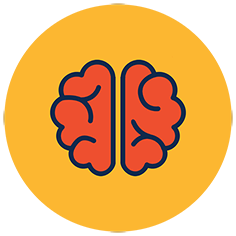## Modular Arithmetic

### Overview

beginner
Mathematics

This course has been put together to help our players develop a strong foundation in the mathematics of which all public key cryptography is built upon. At the heart of modular arithmetic, we are working with familiar operations like addition, multiplication and exponentiation.

However, unlike the integers which just get bigger and bigger, modular arithmetic instead "wraps around" and returns back to zero. Think about clocks, and that how after 23:59 we reach 00:00 instead of 24:00. This introduces subtle changes from the mathematics of our childhood and it's worth taking the time to get used to these differences.

Mathematics can be an intimidating subject, and for many people, a deep dive into cryptography might be the first time someone is really motivated to start working with new areas of mathematics. As well as working on these challenges, if you're finding some of these topics challenging, come chat with us (linked in the side bar) and talk with the community.

For those who already have a strong maths background, these challenges instead are a great way to focus on the kinds of problems you'll be solving within cryptography. Generally, you'll be drawing from your experience of number theory, group theory and later with elliptic curves, algebraic geometry!

For now let's start on some fun challenges to get things moving and very soon we'll be breaking real-world cryptography with our newly developed maths muscles!14827 Players Solving2326 Players Completed395 Points to Earn

### Contents

 Category Challenge Points General - Mathematics Greatest Common Divisor 15 General - Mathematics Extended GCD 20 General - Mathematics Modular Arithmetic 1 20 General - Mathematics Modular Arithmetic 2 20 General - Mathematics Modular Inverting 25 Mathematics - Modular Math Quadratic Residues 25 Mathematics - Modular Math Legendre Symbol 35 Mathematics - Modular Math Modular Square Root 35 Mathematics - Modular Math Chinese Remainder Theorem 40 Mathematics - Brainteasers Part 1 Adrien's Signs 80 Mathematics - Brainteasers Part 1 Modular Binomials 80### Level UpYou are now level Current level